# Polynomials class 10 notes

In this page we will explain the topics for the chapter 2 of Polynomials Class 10 Maths.We have given quality Polynomials Class 10 Notes to explain various things so that students can benefits from it and learn maths in a fun and easy manner, Hope you like them and do not forget to like , social share and comment at the end of the page.
Table of Content

## Introduction

• We have studied polynomial expression in one variable and their degrees in the previous classes.
• Now we know that the highest power of x in p(x) is called the degree of the polynomial p(x).A polynomial of degree 1 is called a linear polynomial , degree 2 is called quadratic polynomial,degree 3 is called a cubic polynomial.
• If p(x) is a polynomial in x, and if k is any real number, then the value obtained by replacing x by k in p(x), is called the value of p(x) at x = k, and is denoted by p(k).A real number k is said to be a zero of a polynomial p(x), if p(k) = 0
• Check out Polynomial expression Degree,Value,Zeroes
• for the details on the above
• Polynomials Addition ,Subtraction ,Multiplication and division were also learned. Check out below links
Multiplying And Dividing Polynomials
• Factoring of Polynomials can be done using grouping,split mid-term method,identity method. This we also learned in earliar classes.Check out How to factor polynomials for details
• This chapter we will focussing on finding the relationship between the coefficients and Zeroes of the polynomials expression. We will also study the division algorithm for polynomial

## Geometric Meaning of the Zero's of the polynomials

• Lets us assume y= p(x) where p(x) is the polynomial of any form.
• Now we can plot the equation y=p(x) on the Cartesian plane by taking various values of x and y obtained by putting the values. The plot or graph obtained can be of any shapes
• The zero's of the polynomial are the points where the graph meet x axis in the Cartesian plane. If the graph does not meet x axis ,then the polynomial does not have any zero's.
• Let us take some useful polynomial , shapes and zeroes obtained on the Cartesian plane

### Linear polynomial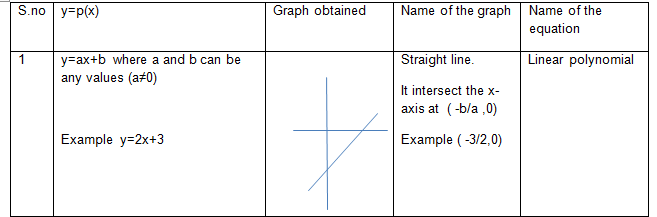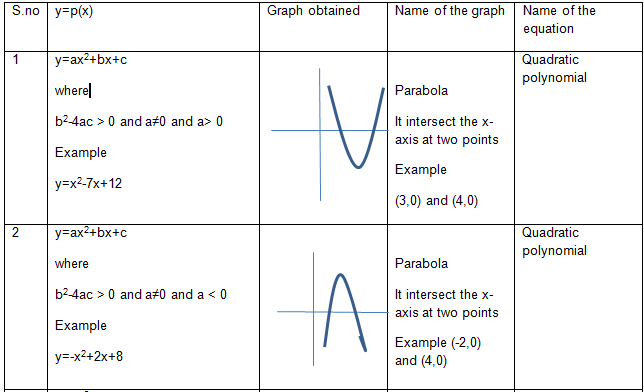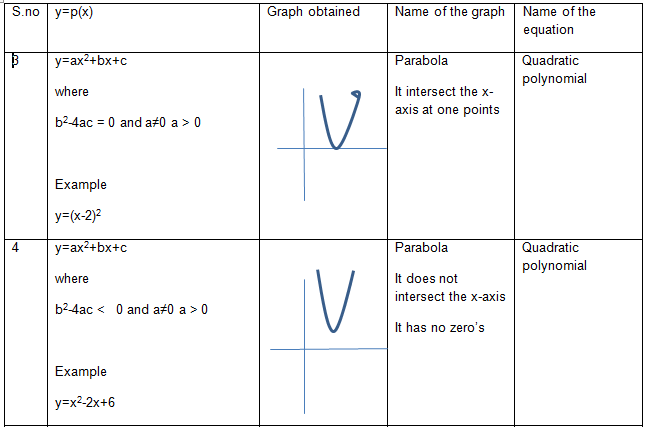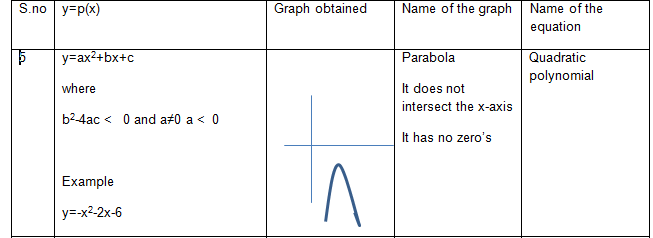### Cubic Polynomial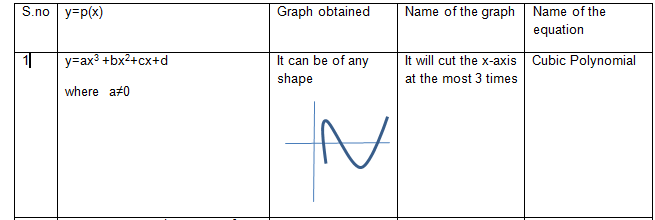### N degree Polynomial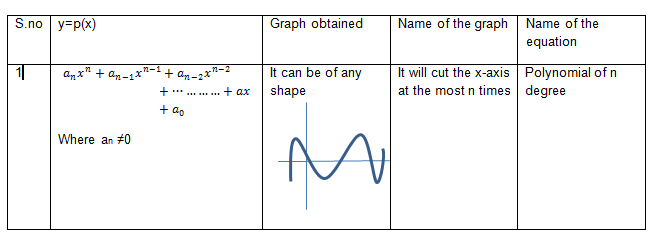More facts about the geometric shape of the Polynomials
• If the degree n of a polynomial is even, then the arms of the graph are either both up or both down. This can be seen in case of Quadratic polynomials
• If the degree n is odd, then one arm of the graph is up and one is down.This can be seen in case of cubic polynomials
• If the leading coefficient $a_n$ is positive, the right arm of the graph is up.
• If the leading coefficient $a_n$ is negative, the right arm of the graph is down
These above points can also be applied to all the example polynomial given up.
These points will help in roughly drawing the graph of any polynomial

## Relation between coefficient and zero's of the Polynomial

• Now that we know about the Geometrical meaning of the Zeros of the Polynomials, lets us the check the relation between the coefficient and zero's of the Polynomials.
• This are very useful formula and it can be used in many places.
• Class 10 Polynomial Syllabus includes relation for Linear ,Quadratic and Cubic Polynomials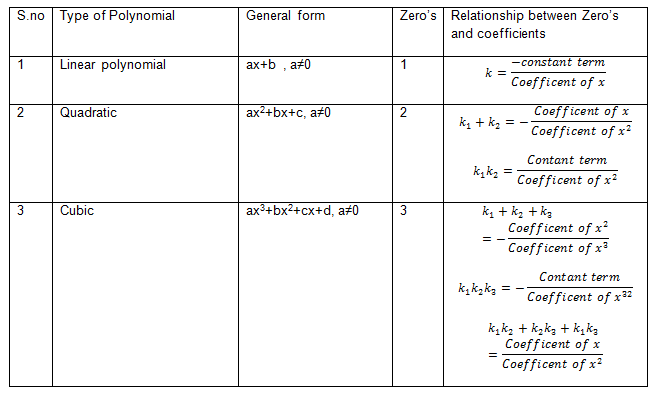Extra Note
For a quatric polynomial
$p(x) = ax^4 + bx^3 + cx^2 + dx + e$
If $\alpha,\ \beta, \ \gamma\ and\ \delta$ are the zeroes of the polynomial p(x)
Then below relationship exists
$\alpha+\ \beta+\ \gamma+\ \delta=\ \frac{-b}{a}$
$\left(\alpha+\ \beta\right)\left(\gamma+\ \delta\right)+\ \left(\alpha\beta\right)+\ \left(\gamma\delta\right)=\ \frac{c}{a}$
$(\alpha+\ \beta)\ \gamma\delta+\left(\gamma+\ \delta\right)\alpha\beta=\ -\frac{d}{a}$
$\alpha\beta\gamma\delta=\ \frac{e}{a}$

## Formation of polynomial when the zeros are given

Now if the zeroes are given , we can find the polynomials based on that also. There will be multiple polynomial having same zeroes. We can just find one of basic one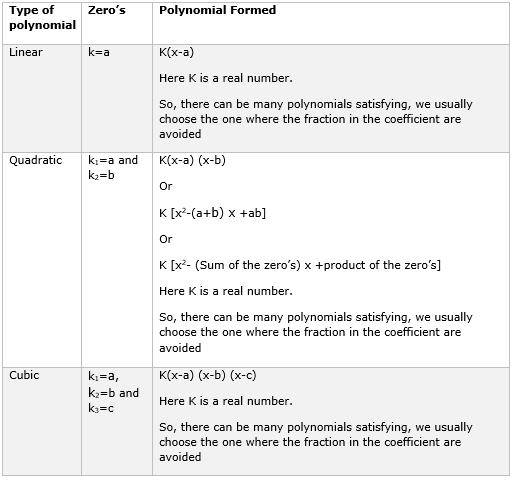## Division algorithm for Polynomial

Let's p(x) and q(x) are any two polynomials with q(x) ≠ 0 ,then we can find polynomial s(x) and r(x) such that
P(x)=s(x) q(x) + r(x)
Where r(x) can be zero or degree of r(x) < degree of g(x)

Steps to divide a polynomial by another polynomial
1. Arrange the term in decreasing order in both the polynomial
2. Divide the highest degree term of the dividend by the highest degree term of the divisor to obtain the first term,
3. Similar steps are followed till we get the reminder whose degree is less than of divisor

Example-1
Divide P(x) by q(x)
$P(x)=x^4 +x +1$
$q(x)=x+1$

Solution
Following the step outlined above,here is the division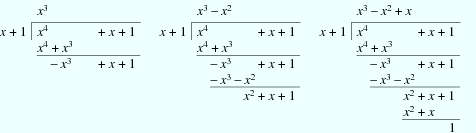So $q(x)=x^3-x^2+x$
r(x)=1

So
$x^4 +x +1=(x+1)(x^3-x^2+x)+1$

### Quiz Time

Question 1 What are zeros of the quadratic polynomial x2 + 3x + 2 ?
A. 3,-2
B. -3,2
C. 3,2
D. -3,-2
Question 2 If p, q, r are the zeroes of the cubic polynomial ax3 + bx2 + cx + d, then ?
A. p+q+r=-b/a
B. pqr=-a/d
C. pq+qr+pr=-c/d
D. none of these
Question 3 Given that two of the zeroes of the cubic polynomial ax3 + bx2+ cx + d are 0, the third zero is .
A. b/a
B. d/a
C. c/a
D. -b/a
Question 4 If the zeroes of the quadratic polynomial ax2+bx +c, where c ≠ 0, are equal then
A. c and a have same sign
B. c and b have same sign
C. c and a have opposite sign
D. c and b have opposite sign
Question 5 Which one is not the zero of polynomial 3x3 - 5x2 - 11x - 3 ?
A. 3
B. -1
C.-1/3
D. 4
Question 6 Write a polynomial function in standard form with zeros at 5, -4, and -3?
A. p(x)=x3 + 2x2 -23x+7
B. p(x)=x3 - 60x2 +2x-23
C. p(x)=x3 + 2x2 +23x-1
D. p(x)=x3 + 2x2 -23x-60

## Summary

Here is the Polynomials Class 10 Notes Summary
• The zeroes of a polynomial p(x) are the x-coordinates of the points, where the graph of y = p(x) intersects the x -axis. If it does not cut x-axis ,then it has no zeroes
• A quadratic polynomial can have at most 2 zeroes and a cubic polynomial can have at most 3 zeroes
• The Sum and Product of the roots of the quadratic polynomials $ax^2 + bx+ c$ is
$\alpha + \beta = -\frac {b}{a}$ and $\alpha \beta= \frac {c}{a}$
• The relationshio between the roots of the cubic polynomials $ax^3 + bx^2 +cx + d$ are
$\alpha + \beta + \gamma = -\frac {b}{a}$ , $\alpha \beta + \beta \gamma + \alpha \gamma= \frac {c}{a}$ and $\alpha \beta \gammm= -\frac {d}{a}$Go back to Class 10 Main Page using below links

### Practice Question

Question 1 What is $1 - \sqrt {3}$ ?
A) Non terminating repeating
B) Non terminating non repeating
C) Terminating
D) None of the above
Question 2 The volume of the largest right circular cone that can be cut out from a cube of edge 4.2 cm is?
A) 19.4 cm3
B) 12 cm3
C) 78.6 cm3
D) 58.2 cm3
Question 3 The sum of the first three terms of an AP is 33. If the product of the first and the third term exceeds the second term by 29, the AP is ?
A) 2 ,21,11
B) 1,10,19
C) -1 ,8,17
D) 2 ,11,20